2019-01-23 13:45:57 qq_35114061 阅读数 875
• ###### MATLAB图像处理

全面系统的学习MATLAB在图像处理中的应用

19886 人正在学习 去看看 魏伟

# 图像处理 – 识别刻度

     需求:
1.从图片文件夹中挑选出清晰度最高的一张图片，删除剩余；
2.算法识别最左端的刻度线，以中间直线为基准，从左向右扫描检测区域，
识别每一条刻度的边缘，一直扫描到不再有灰度的变化为止，根据刻度
线条的个数得到刻度值，如：测到三根刻度线，两根线之间为 0.5mm,与
10 作差，取绝对值，|10-（3-1）*0.5）|=9mm。
3.保存处理的图片，并把测量结果显示到图片上。


## 思路：

1. 使用方差进行清晰度比较；
2. 二值化生成0,1组成txt 文件，可以通过数字图像轮廓计算获得测量值；

## 第一步：（计算文件夹中所用图片方差）：

 /// <summary>
/// 计算图片清晰度
/// </summary>
/// <param name="bmp">目标图片</param>
/// <returns>返回方差值</returns>
public static int CalculateDefinition(Bitmap bmp)
{
//灰度值
List<byte> list = new List<byte>();
//平均值
int average = 0;
for (int i = 0; i < bmp.Width; i++)
{
for (int j = 0; j < bmp.Height; j++)
{
//获取该点的像素的RGB的颜色
Color color = bmp.GetPixel(i, j);
byte gray = (byte)(0.299f * color.R + 0.587f * color.G + 0.114f * color.B);
average += gray;
Color newColor = Color.FromArgb(gray, gray, gray);
}
}
//获取平均值
average = average / (bmp.Width * bmp.Height);
//计算方差
int sum = 0;
for (int k = 0; k < list.Count; k++)
{
sum += (list[k] - average) * (list[k] - average);
}
sum = sum / (bmp.Width * bmp.Height);
return sum;
}


## 第二步（选中的图片进行二值化）

        /// <summary>
/// 二值化图像
/// </summary>
/// <param name="bmp"></param>
/// <returns></returns>
public static unsafe Bitmap Binaryzation(Bitmap bmp)
{
BitmapData dstData = bmp.LockBits(new Rectangle(0, 0, bmp.Width, bmp.Height), ImageLockMode.ReadWrite, bmp.PixelFormat);
byte* data = (byte*)(dstData.Scan0);
//将图像转换为0,1二值得图像;
int step = dstData.Stride;
int means = getThreshold(data, bmp.Height * step);
for (int y = 0; y < bmp.Height; y++)
{
for (int x = 0; x < bmp.Width * 3; x += 3)
{
if (data[y * step + x + 2] > means)
data[y * step + x]
= data[y * step + x + 1]
= data[y * step + x + 2]
= 255;
else
data[y * step + x]
= data[y * step + x + 1]
= data[y * step + x + 2]
= 0;
}
}
bmp.UnlockBits(dstData);
return bmp;
}
/// <summary>
/// 图像二值化 获取阀值
/// </summary>
/// <param name="inPixels"></param>
/// <param name="length">height * Stride</param>
/// <returns></returns>
private static unsafe int getThreshold(byte* inPixels, int length)
{
int inithreshold = 127;
int finalthreshold = 0;
List<int> temp = new List<int>();
for (int index = 0; index < length; index += 3)
{
}
List<int> sub1 = new List<int>();
List<int> sub2 = new List<int>();
int means1 = 0, means2 = 0;
while (finalthreshold != inithreshold)
{
finalthreshold = inithreshold;
for (int i = 0; i < temp.Count(); i++)
{
if (temp[i] <= inithreshold)
{
}
else
{
}
}
means1 = getMeans(sub1);
means2 = getMeans(sub2);
sub1.Clear();
sub2.Clear();
inithreshold = (means1 + means2) / 2;
}
return finalthreshold;
}
/// <summary>
/// 图像二值化 获取Means
/// </summary>
/// <param name="data"></param>
/// <returns></returns>
private static int getMeans(List<int> data)
{
int result = 0;
int size = data.Count();
foreach (int i in data)
{
result += i;
}
return (result / size);
}


## 第三步（选中的图片转化为只包含01数组并写成txt文件）

 public static byte[] getArray(Bitmap bp)
{
byte[] data = new byte[bp.Width * bp.Height];
int g = 0;
for (int i = 0; i < bp.Height; i++)
{
for (int j = 0; j < bp.Width; j++)
{
//获取该点的像素的RGB的颜色
Color color = bp.GetPixel(j, i);
//data[i, j] = color.R;

if (color.R == 255)
{
data[g] = 1;
}
else
{
data[g] = 0;
}
g++;
}
}
return data;
}
public static void arrayTotextFile(string filePath, byte[] newList1, int length)
{
List<byte> list = new List<byte>();
//创建一个文件流，用以写入或者创建一个StreamWriter
FileStream fs = new FileStream(filePath, FileMode.OpenOrCreate, FileAccess.Write);
StreamWriter sw = new StreamWriter(fs);
sw.Flush();
// 使用StreamWriter来往文件中写入内容
sw.BaseStream.Seek(0, SeekOrigin.Begin);
for (int i = 0; i < newList1.Length; i++)
{
if (i != 0 && i % length == 0)
{
sw.Write(newList1[i] + Environment.NewLine);
}
else
{
sw.Write(newList1[i]);
}
}
//关闭此文件t
sw.Flush();
sw.Close();
fs.Close();
}


## 第三步：计算测量值

    /// <summary>
/// 获得字符连续出现次数
/// </summary>
public static int getCharOccurrencesCount(string str)
{
int effectiveCount = 0;
Regex reg = new Regex(@"(.)(\1)+");
MatchCollection matchs = reg.Matches(str);
if (matchs != null)
{
foreach (Match m in matchs)
{
string mValue = m.Value;
if (mValue.Contains("0"))
{
effectiveCount++;
}
}
}
return effectiveCount;
}
/// <summary>
/// 采集N次数据，挑选次数最多的为最终的刻度
/// </summary>
/// <param name="finallCount">记录多次采集刻度线个数</param>
/// <returns></returns>
public static int getCount(List<int> finallCount)
{
int count = 1;

Dictionary<int, int> map = new Dictionary<int, int>();
for (int i = 0; i < finallCount.Count; i++)
{
count = 1;
for (int j = i + 1; j < finallCount.Count(); j++)
{
if (finallCount[i] == finallCount[j])
{
count++;// 次数+1
finallCount.RemoveAt(j);
j--;// 这里是重点，因为集合remove（）后，长度改变了，对应的下标也不再是原来的下标
}
}
Console.WriteLine(finallCount[i] + "出现次数" + count);
}

//降序排序
var dicSort = from objDic in map orderby objDic.Value descending select objDic;

foreach (KeyValuePair<int, int> kvp in dicSort)
{
//Response.Write(kvp.Key + "：" + kvp.Value + "<br />");
Console.WriteLine(kvp.Key + "：" + kvp.Value);
count = kvp.Key;
break;
}
return count;
}


## 效果展示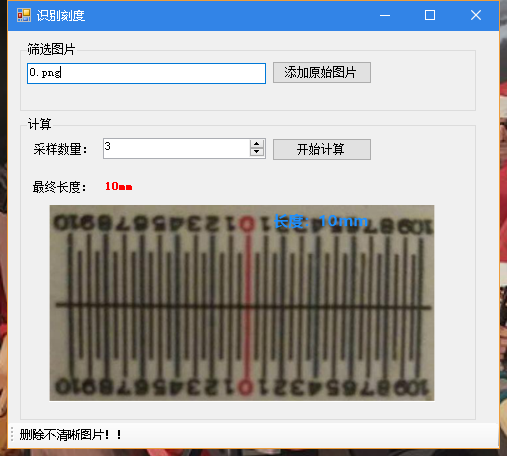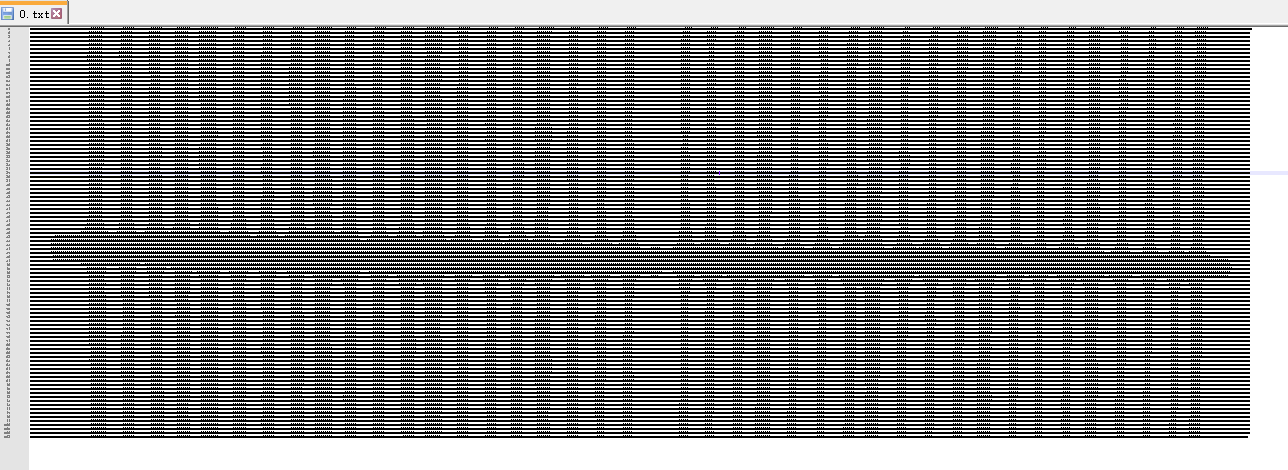2019-08-25 10:40:47 weixin_43585712 阅读数 43
• ###### MATLAB图像处理

全面系统的学习MATLAB在图像处理中的应用

19886 人正在学习 去看看 魏伟

# 1.用霍夫变换检测直线和圆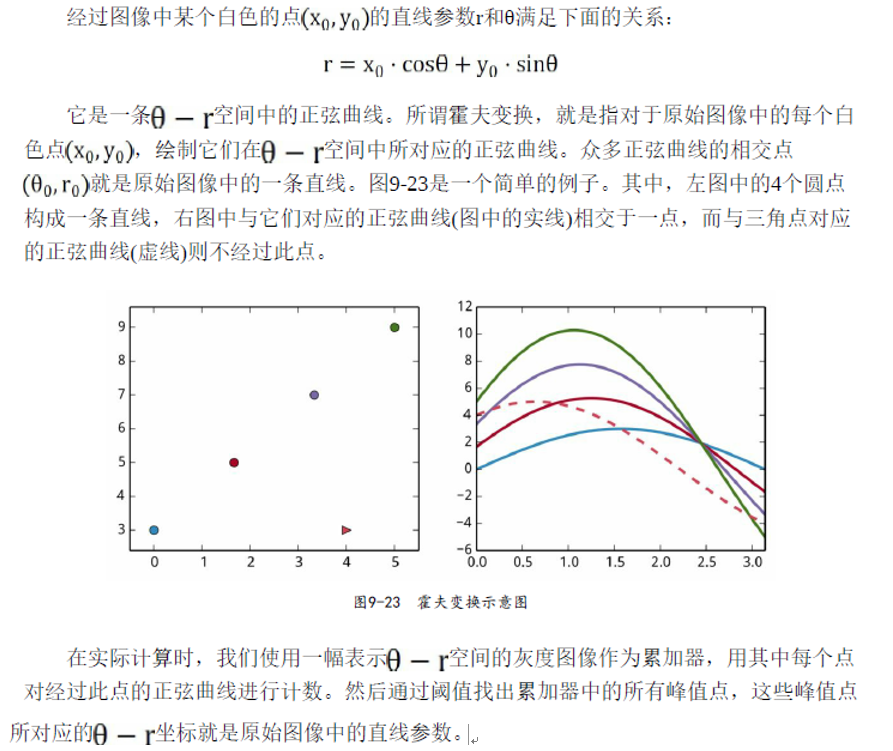OpenCV提供了如下三种霍夫变换相关的函数：
HoughLines：检测图像中的直线。
HoughLinesP：检测图像中的直线段。
HoughCircles：检测图像中的圆。

HoughLinesP(image, rho, theta, threshold[, lines[, minLineLength[, maxLineGap]]])

image参数为进行直线检测的图像
rho和theta参数分别为累加器中每个点所表示的r和θ的大小。其中rho的单位是像素点，而theta是以弧度表示的角度。

threshold参数是 在累加器中寻找峰值时所使用的阈值，即只有大于此值的峰值点才被当作与某条直线相对 应。

maxLineGap参数则指定线段的最大间隙。当有多条线段共线时，间隙小于此值 线段将被合并为一条线段。


import cv2
import numpy as np
import matplotlib.pyplot as pl
pl.rcParams["font.family"] = "SimHei"#直接修改配置字典，设置默认字体

#输入图像进行边缘检测,得到一个二值图像
#（输入图像，阈值1，阈值2）阈值越小，从图像中检测的细节越多
img_binary = cv2.Canny(img, 100, 255)
#（被检测的图像，累加器参数，，在累加器中寻找峰值时所使用的阈值，线段的最小长度，线段的最大间隙）
#HoughLinesP()的返回值是一个形状为(N,1,4)的数组，其中N为线段数，第二轴的4个元素为线段的起点和终点：x0、y0、x1、y1。
threshold=96, minLineLength=33,maxLineGap=4)#(85,1,4)

fig, ax = pl.subplots(figsize=(8, 6))
pl.imshow(img, cmap="gray")

from matplotlib.collections import LineCollection
lc = LineCollection(lines.reshape(-1, 2, 2))
ax.axis("off")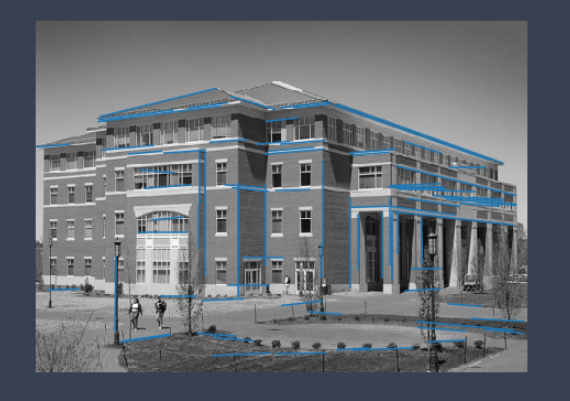method参数为圆形检测的算法，目前OpenCV中只实现了一种检测算法：CV_HOUGH_GRADIENTP
dp参数和直线检测中的rho参数类似，决定了检测的精度， dp=1时累加器的分辨率和输入图像相同，而dp=2时累加器的分辨率为输入图像的一半。
minDist参数是检测到的所有圆的圆心之间的最小距离，当它过小时会检测出很多近似的圆形，若过大则可能会漏掉一些结果。
param1参数相当于边缘检测Canny()的第二个阈值，Canny()的第一个阈值自动设置为它的一半。
param2参数是累加器上的阈值，它的值越小检测出的圆形越多。


import cv2
import numpy as np
import matplotlib.pyplot as pl
pl.rcParams["font.family"] = "SimHei"#直接修改配置字典，设置默认字体

#为了获得较好的边缘检测结果，调用GaussianBlur()对图像进行模糊处理。
img_blur = cv2.GaussianBlur(img, (0, 0), 1.8)
x, y, r = circles.T#(1,24,3)变成(24,3)。第一列给x,2-y,3-r

fig, ax = pl.subplots(figsize=(8, 6))
pl.imshow(img, cmap="gray")

from matplotlib.collections import EllipseCollection
#使用EllipseCollection快速绘制多个圆形
ec = EllipseCollection(widths=2*r, heights=2*r, angles=0, units="xy",
facecolors="none", edgecolors="red",transOffset=ax.transData, offsets=np.c_[x, y])
ax.axis("off")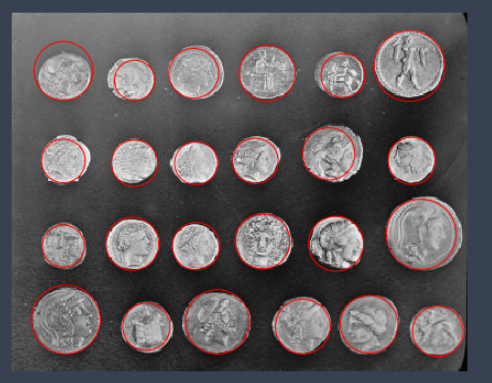# 2.图像分割

## 2.1Mean-Shift算法

pyrMeanShiftFiltering()使用Mean-Shift算法对图像进行分割。它的调用参数如下：

pyrMeanShiftFiltering(src, sp, sr[, dst[, maxLevel[, termcrit]]])

fig, axes = pl.subplots(1, 3, figsize=(9, 3))
srs = [20, 40, 80]
for ax, sr in zip(axes, srs):
img2 = cv2.pyrMeanShiftFiltering(img, sp=20, sr=sr, maxLevel=1)
ax.imshow(img2[:,:,::-1])
ax.set_axis_off()
ax.set_title("sr = {}".format(sr))

fig.subplots_adjust(0.02, 0, 0.98, 1, 0.02, 0)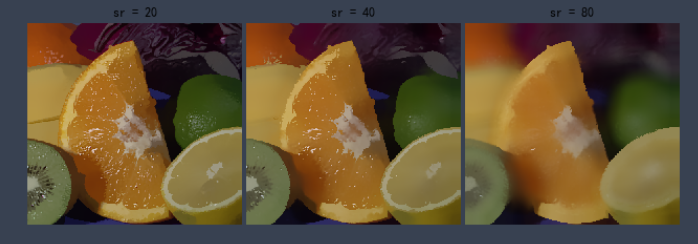## 2.2分水岭算法(重要）

watershed()实现此算法，它的调用形式如下：
watershed(image, markers)

   image参数是需要进行分割处理的图像，它必须是一个3通道8位图像。
markers参数是一个32位整数数组，其大小必须和image相同。 markers中值大于0的点构成初始灌溉区域，其值可以理解为水的颜色。
调用watershed()之后，markers中几乎所有的点都将被赋值为某个初始区域的值，而在两个区域的境界线上的点将被赋值为-1。

import cv2
import numpy as np
import matplotlib.pyplot as pl
pl.rcParams["font.family"] = "SimHei"#直接修改配置字典，设置默认字体
fig, axes = pl.subplots(1, 2, figsize=(10, 6))
a0,a1=axes,axes
img_gray = cv2.cvtColor(img, cv2.COLOR_BGR2GRAY)
a0.imshow(img_gray,cmap='gray')
#先调用blur()对药丸的灰度图像进行模糊处理，这样可以有效消除噪声，减少局域最值的个数。
img_gray = cv2.blur(img_gray, (15, 15))

_, img_binary = cv2.threshold(img_gray, 150, 255, cv2.THRESH_BINARY)

#局域最亮像素就是比周边临近的像素都亮的像素，使用dilate()对灰度图像进行膨胀处
#理，将每个像素都设置为邻近像素中的最大值，然后与原始图像中的值比较，如果值保持
#不变，该像素即为局域最亮像素。
peaks = img_gray == cv2.dilate(img_gray, np.ones((7, 7)), 1)
peaks =peaks*img_binary#非零值为局部最亮，其余为0值

peaks[1, 1] = True #设置初始值

from scipy.ndimage import label
markers, count = label(peaks) #对区域进行编号
marks=cv2.watershed(img, markers)#边界值为-1
img2=img
img2[marks == -1] = [0,0,255]
a1.imshow(img2)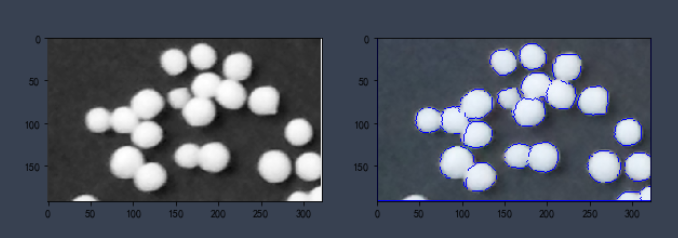# 3.SURF特征匹配

SURF是一种快速提取图像特征点的算法，它所提取的图像特征具有缩放和旋转的不变性，而且它对图像的灰度变化也不敏感。对SURF算法的详细介绍还有待研究，这里只简单地介绍OpenCV中SURF类的用法。

import cv2
import numpy as np
import matplotlib.pyplot as pl
pl.rcParams["font.family"] = "SimHei"#直接修改配置字典，设置默认字体

#然后创建SURF()对象，并设置hessianThreshold和nOctaves参数，这两个参数为计算关键点的参数，
#hessianThreshold越大则关键点的个数越少，nOctaves越大则关键点的尺寸范围越大。
surf = cv2.xfeatures2d.SURF_create (2000, 2)
#调用detect()方法从灰度图像中检测出关键点。
#它返回一个列表key_points，其中每个元素都是一个保存关键点信息的KeyPoint对象。
key_points1 = surf.detect(img_gray1)
#根据关键点的size属性按照从大到小的顺序排列关键点
key_points1.sort(key=lambda x:x.size, reverse=True)

#灰度图像通过cvtColor()转换成灰色的RGB格式的图像
img_color1 = cv2.cvtColor(img_gray1, cv2.COLOR_GRAY2RGB)
#调用drawKeypoints()可以在图像上绘制关键点
#（绘制的图像，关键点（前25个），输出图像，颜色设置，绘图标志）
#绘图标志：。图中红色圆圈的大小显示关键点的size属性，而半径线段的方向显示了关键点的方向属性angle。
cv2.drawKeypoints(img_color1, key_points1[:25], img_color1, color=(255, 0, 0),
flags=cv2.DRAW_MATCHES_FLAGS_DRAW_RICH_KEYPOINTS)
pl.imshow(img_color1)

#调用SURF.compute()计算关键点列表key_points1对应的特征向量features1
_, features1 = surf.compute(img_gray1, key_points1)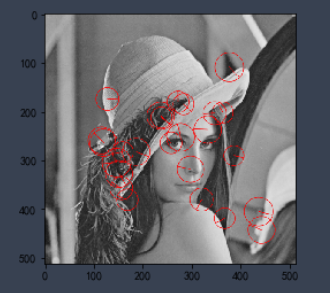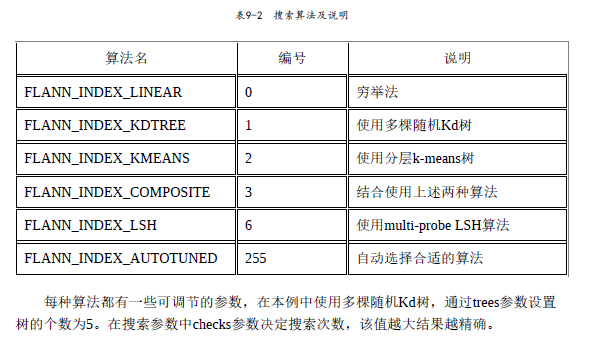"关键点数很多，可以使用OpenCV提供的FlannBasedMatcher寻找匹配的特征向量。"
import cv2
import numpy as np
import matplotlib.pyplot as pl
pl.rcParams["font.family"] = "SimHei"#直接修改配置字典，设置默认字体
"1.准备两幅图像"

"2.直接计算关键点以及与之对应的特征向量"
key_points1, features1 = surf.detectAndCompute(img_gray1, None)
key_points2, features2 = surf.detectAndCompute(img_gray2, None)

"3.调用匹配算法"
FLANN_INDEX_KDTREE = 1
#创建FlannBasedMatcher对象时传递两个字典index_params和search_params，分别设置其索引参数和搜索参数。
index_params = dict(algorithm=FLANN_INDEX_KDTREE, trees=5)
search_params = dict(checks=100)
fbm = cv2.FlannBasedMatcher(index_params, search_params)
#调用knnMatch()对features1中的每个特征向量在features2中搜索k个最近的特征向量。
#这里设置参数k为1，只搜索最接近的特征向量。
match_list = fbm.knnMatch(features1, features2, k=2) #嵌套列表
matchesMask = [[0,0] for i in range(len(match_list))]
# ratio test as per Lowe's paper
for i,(m,n) in enumerate(match_list):
if m.distance < 0.8*n.distance:

img = cv2.drawMatchesKnn(img_gray1,key_points1,img_gray2,key_points2,match_list,None,**draw_params)
fig, axes = pl.subplots(1, 1, figsize=(15, 8))
axes.imshow(img[:,:,::-1])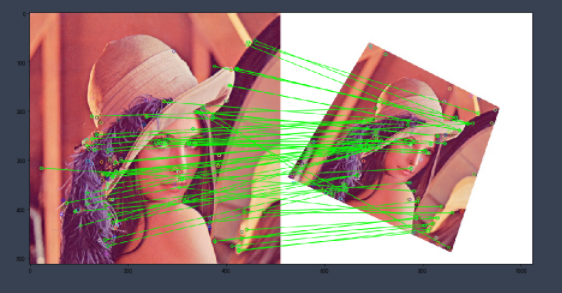2018-08-25 22:04:16 qq_40962368 阅读数 5338
• ###### MATLAB图像处理

全面系统的学习MATLAB在图像处理中的应用

19886 人正在学习 去看看 魏伟

# 霍夫变换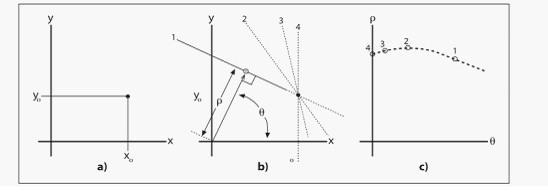（c）所示为这四条直线在极坐标参数空间可以表示为四个点。

SHT就是上述的在极坐标空间进行参数表示的方法，而PPHT是SHT的改进，它是在一定的范围内进行霍夫变换，从而减少计算量，缩短计算时间。

### （一）标准霍夫变换

• 第一个参数，是需要进行检测的灰度图
• 第二、三参数分别是的精确度，可以理解为步长。
• 第四个参数为阈值T，认为当累加器中的值高于所设定的阈值T时，才认为是一条直线。
# 标准霍夫变换
house = cv2.cvtColor(img, cv2.COLOR_BGR2GRAY)  # 获取灰度图
edges = cv2.Canny(house, 50, 200)
lines = cv2.HoughLines(edges, 1, np.pi/180, 260)  # 霍夫变换返回的就是极坐标系中的两个参数  rho和theta
print(np.shape(lines))
lines = lines[:, 0, :]  # 将数据转换到二维
for rho, theta in lines:
a = np.cos(theta)
b = np.sin(theta)
# 从图b中可以看出x0 = rho x cos(theta)
#               y0 = rho x sin(theta)
x0 = a*rho
y0 = b*rho
# 由参数空间向实际坐标点转换
x1 = int(x0 + 1000*(-b))
y1 = int(y0 + 1000*a)
x2 = int(x0 - 1000*(-b))
y2 = int(y0 - 1000*a)
cv2.line(img, (x1, y1), (x2, y2), (0, 0, 255), 1)
cv2.imshow('img', img)
cv2.imshow('edges', edges)
cv2.waitKey(0)
cv2.destroyAllWindows()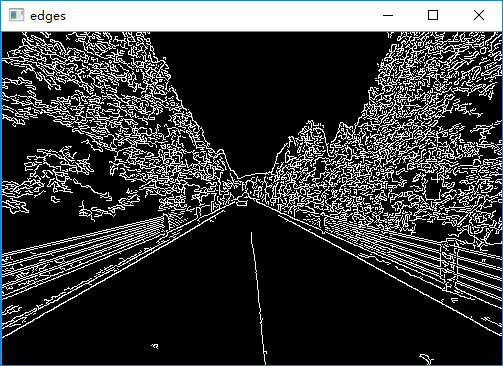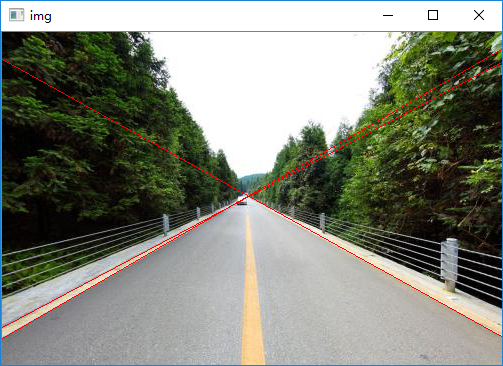### （二）渐进概率式霍夫变换

# 渐进概率式霍夫变换
gray = cv2.cvtColor(img, cv2.COLOR_BGR2GRAY)
edges = cv2.Canny(gray, 50, 250)
lines = cv2.HoughLinesP(edges, 1, np.pi/180, 30, minLineLength=60, maxLineGap=10)
lines = lines[:, 0, :]
for x1, y1, x2, y2 in lines:
cv2.line(img, (x1, y1), (x2, y2), (0, 255, 0), 1)
cv2.imshow('img', img)
cv2.waitKey(0)
cv2.destroyAllWindows()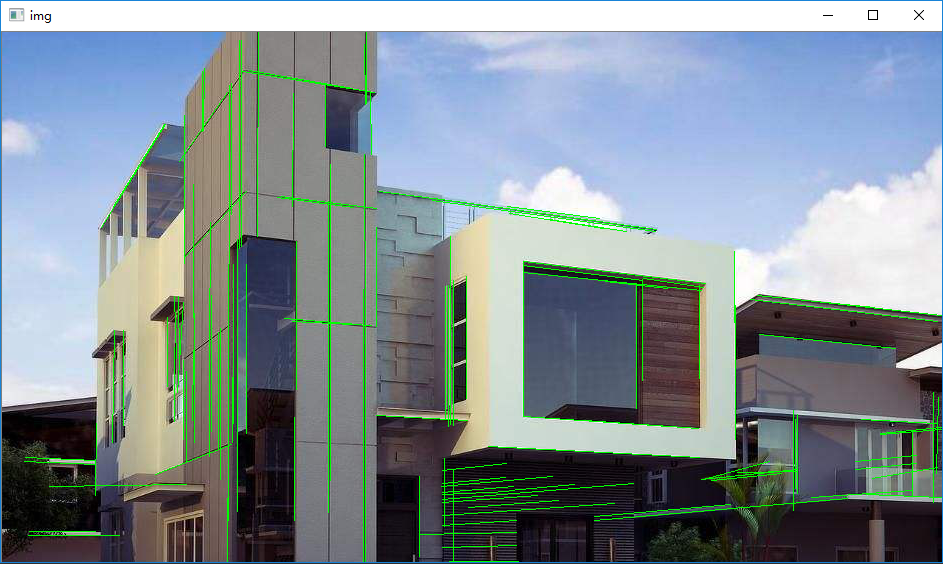### （三）利用霍夫变换检测圆环

param1和param2就是的精确度，最后两个参数是所要检测的圆的最大最小半径，不能盲目的检测，否则浪费时间和空间。输出就是三个参数空间矩阵。

img = cv2.imread('yinzhang.jpg')
gray = cv2.cvtColor(img, cv2.COLOR_BGR2GRAY)
cv2.imshow('img_yuantu', img)
gaussian = cv2.GaussianBlur(gray, (3, 3), 0)
circles = circles1[0, :, :]
circles = np.uint16(np.around(circles))
for i in circles[:]:
cv2.circle(img, (i, i), i, (0, 255, 0), 3)
cv2.circle(img, (i, i), 2, (255, 0, 255), 10)
cv2.imshow('img', img)
cv2.waitKey(0)
cv2.destroyAllWindows()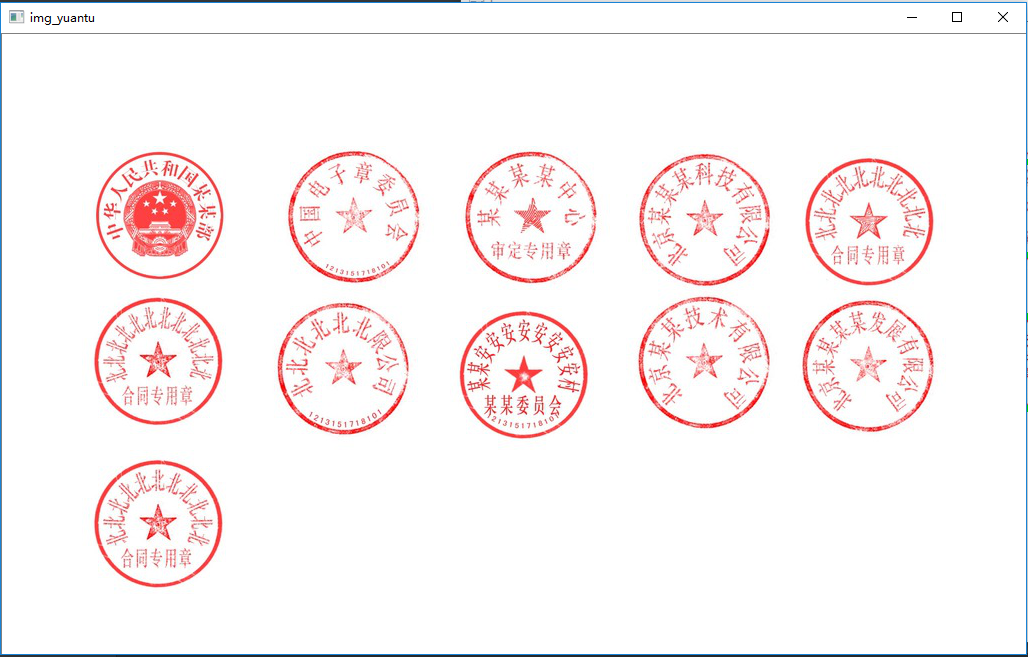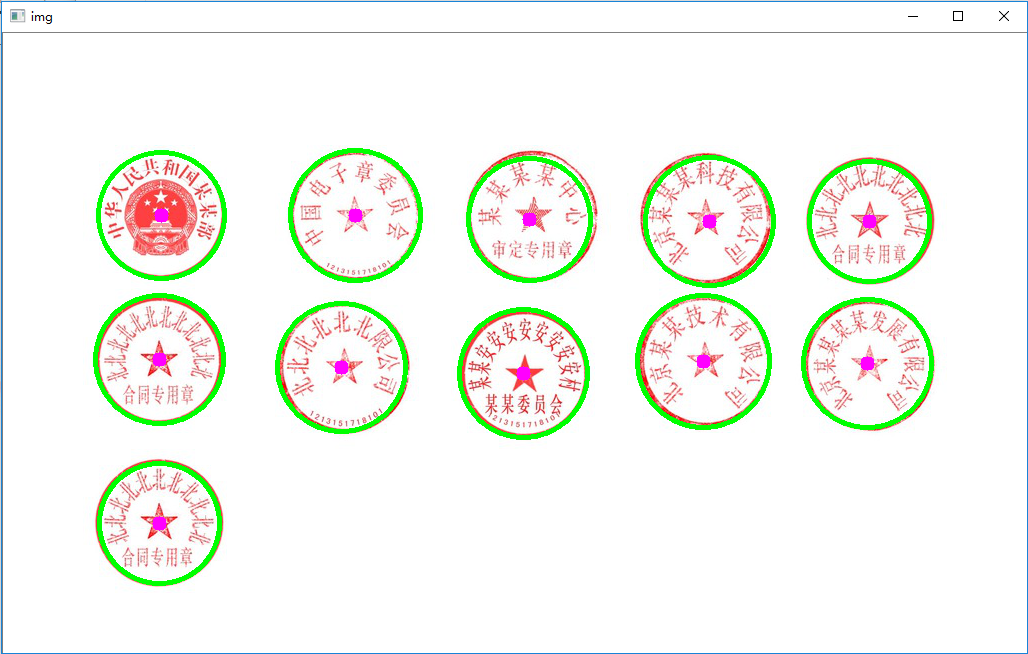2019-10-21 15:21:49 qq_24624539 阅读数 183
• ###### MATLAB图像处理

全面系统的学习MATLAB在图像处理中的应用

19886 人正在学习 去看看 魏伟

1.霍夫变换(Hough Transform) 霍夫变换是图像处理中从图像中识别几何形状的基本方法之一，应用很广泛，也有很多改进算法。主要用来从图像中分离出具有某种相同特征的几何形状（如，直线，圆等）。最基本的霍夫变换是从黑白图像中检测直线(线段)。

2.Hough变换的原理是将特定图形上的点变换到一组参数空间上，根据参数空间点的累计结果找到一个极大值对应的解，那么这个解就对应着要寻找的几何形状的参数（比如说直线，那么就会得到直线的斜率k与常熟b，圆就会得到圆心与半径等等）

3.霍夫线变换是一种用来寻找直线的方法。用霍夫线变换之前, 首先需要对图像进行边缘检测的处理，也即霍夫线变换的直接输入只能是边缘二值图像。

4.霍夫直线检测的具体原理参见：

https://blog.csdn.net/ycj9090900/article/details/52944708

http://www.opencv.org.cn/opencvdoc/2.3.2/html/doc/tutorials/imgproc/imgtrans/hough_lines/hough_lines.html

http://lib.csdn.net/article/opencv/24201#直线检测
#使用霍夫直线变换做直线检测，前提条件：边缘检测已经完成
import cv2 as cv
import numpy as np

#标准霍夫线变换
def line_detection(image):
gray = cv.cvtColor(image, cv.COLOR_RGB2GRAY)
edges = cv.Canny(gray, 50, 150, apertureSize=3)  #apertureSize参数默认其实就是3
cv.imshow("edges", edges)
lines = cv.HoughLines(edges, 1, np.pi/180, 80)
for line in lines:
rho, theta = line  #line存储的是点到直线的极径和极角，其中极角是弧度表示的。
a = np.cos(theta)   #theta是弧度
b = np.sin(theta)
x0 = a * rho    #代表x = r * cos（theta）
y0 = b * rho    #代表y = r * sin（theta）
x1 = int(x0 + 1000 * (-b)) #计算直线起点横坐标
y1 = int(y0 + 1000 * a)    #计算起始起点纵坐标
x2 = int(x0 - 1000 * (-b)) #计算直线终点横坐标
y2 = int(y0 - 1000 * a)    #计算直线终点纵坐标    注：这里的数值1000给出了画出的线段长度范围大小，数值越小，画出的线段越短，数值越大，画出的线段越长
cv.line(image, (x1, y1), (x2, y2), (0, 0, 255), 2)    #点的坐标必须是元组，不能是列表。
cv.imshow("image-lines", image)

#统计概率霍夫线变换
def line_detect_possible_demo(image):
gray = cv.cvtColor(image, cv.COLOR_RGB2GRAY)
edges = cv.Canny(gray, 50, 150, apertureSize=3)  # apertureSize参数默认其实就是3
lines = cv.HoughLinesP(edges, 1, np.pi / 180, 60, minLineLength=60, maxLineGap=5)
for line in lines:
x1, y1, x2, y2 = line
cv.line(image, (x1, y1), (x2, y2), (0, 0, 255), 2)
cv.imshow("line_detect_possible_demo",image)

print(src.shape)
cv.namedWindow('input_image', cv.WINDOW_AUTOSIZE)
cv.imshow('input_image', src)
line_detection(src)
line_detect_possible_demo(src)
cv.waitKey(0)
cv.destroyAllWindows()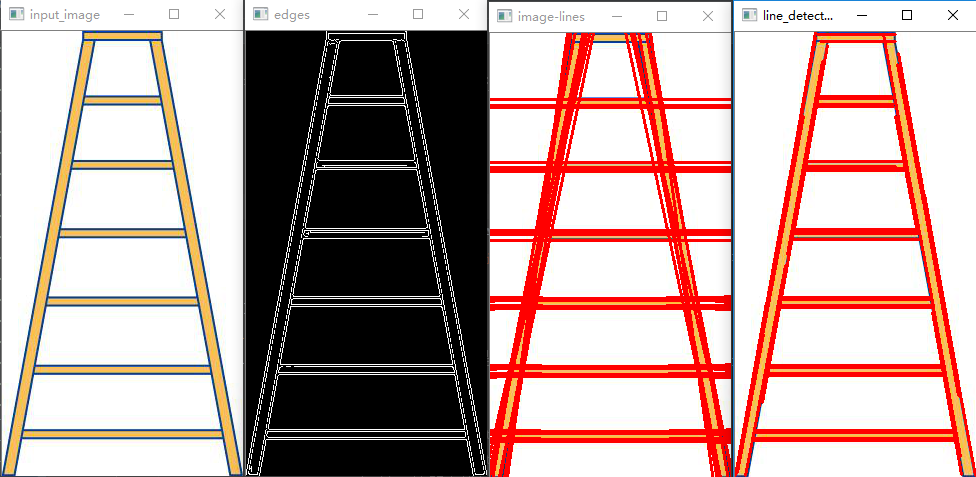1.opencv的HoughLines函数是标准霍夫线变换函数，该函数的功能是通过一组参数对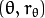的集合来表示检测到的直线，其函数原型为：HoughLines(image, rho, theta, threshold[, lines[, srn[, stn[, min_theta[, max_theta]]]]]) -> lines

image参数表示边缘检测的输出图像，该图像为单通道8位二进制图像。

rho参数表示参数极径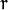以像素值为单位的分辨率，这里一般使用1像素。

theta参数表示参数极角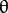以弧度为单位的分辨率，这里使用1度。

threshold参数表示检测一条直线所需最少的曲线交点。

lines参数表示储存着检测到的直线的参数对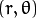的容器 。

srn参数、stn参数默认都为0。如果srn = 0且stn = 0，则使用经典的Hough变换。

min_theta参数表示对于标准和多尺度Hough变换，检查线条的最小角度。

max_theta参数表示对于标准和多尺度Hough变换，检查线条的最大角度。

2.opencv的HoughLinesP函数是统计概率霍夫线变换函数，该函数能输出检测到的直线的端点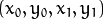，其函数原型为：HoughLinesP(image, rho, theta, threshold[, lines[, minLineLength[, maxLineGap]]]) -> lines

image参数表示边缘检测的输出图像，该图像为单通道8位二进制图像。

rho参数表示参数极径以像素值为单位的分辨率，这里一般使用 1 像素。

theta参数表示参数极角以弧度为单位的分辨率，这里使用 1度。

threshold参数表示检测一条直线所需最少的曲线交点。

lines参数表示储存着检测到的直线的参数对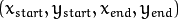的容器，也就是线段两个端点的坐标。

minLineLength参数表示能组成一条直线的最少点的数量，点数量不足的直线将被抛弃。

maxLineGap参数表示能被认为在一条直线上的亮点的最大距离。

2019-03-20 14:17:10 zx_good_night 阅读数 307
• ###### MATLAB图像处理

全面系统的学习MATLAB在图像处理中的应用

19886 人正在学习 去看看 魏伟
import cv2
import numpy as np
from matplotlib import pyplot as plt

__author__ = "zxsuperstar"
__email__ = "zxsuperstar@163.com"

"""

1.霍夫变换(Hough Transform) 霍夫变换是图像处理中从图像中识别几何形状的基本方法之一，

2.Hough变换的原理是将特定图形上的点变换到一组参数空间上，根据参数空间点的累计结果找到一个极大值对应的解，

3.霍夫线变换是一种用来寻找直线的方法。用霍夫线变换之前, 首先需要对图像进行边缘检测的处理，

"""
def line_detection(image):
gray = cv2.cvtColor(image,cv2.COLOR_BGRA2GRAY)
edges = cv2.Canny(gray,50,150,apertureSize=3)
lines = cv2.HoughLines(edges,1,np.pi/180,200)
for line in lines:
rho, theta = line
a = np.cos(theta)
b = np.sin(theta)
x0 = a * rho
y0 = b * rho
x1 = int(x0+1000*(-b))
y1  = int(y0+1000*(a))
x2 = int(x0 - 1000 * (-b))
y2 = int(y0 - 1000 * (a))
cv2.line(image,(x1,y1),(x2,y2),(0,0,255),2)
cv2.imshow("image_line",image)

def line_detect_possible_demo(image):
gray = cv2.cvtColor(image, cv2.COLOR_BGRA2GRAY)
edges = cv2.Canny(gray, 50, 150, apertureSize=3)
lines = cv2.HoughLinesP(edges, 1, np.pi / 180, 100, minLineLength=50,maxLineGap=10)
for line in lines:
x1,y1,x2,y2 = line
cv2.line(image, (x1, y1), (x2, y2), (0, 0, 255), 2)
cv2.imshow("line_detect_possible_demo", image)

if __name__ == "__main__":
src = cv2.imread("t.jpg") #blue green red
# cv2.namedWindow("image", cv2.WINDOW_AUTOSIZE)
# cv2.imshow("image",src)
line_detect_possible_demo(src)
# line_detection(src)
cv2.waitKey(0)
cv2.destroyAllWindows()



1.opencv的HoughLines函数是标准霍夫线变换函数，该函数的功能是通过一组参数对的集合来表示检测到的直线，其函数原型为：HoughLines(image, rho, theta, threshold[, lines[, srn[, stn[, min_theta[, max_theta]]]]]) -> lines

image参数表示边缘检测的输出图像，该图像为单通道8位二进制图像。

rho参数表示参数极径以像素值为单位的分辨率，这里一般使用1像素。

theta参数表示参数极角以弧度为单位的分辨率，这里使用1度。

threshold参数表示检测一条直线所需最少的曲线交点。

lines参数表示储存着检测到的直线的参数对的容器 。

srn参数、stn参数默认都为0。如果srn = 0且stn = 0，则使用经典的Hough变换。

min_theta参数表示对于标准和多尺度Hough变换，检查线条的最小角度。

max_theta参数表示对于标准和多尺度Hough变换，检查线条的最大角度。

2.opencv的HoughLinesP函数是统计概率霍夫线变换函数，该函数能输出检测到的直线的端点，其函数原型为：HoughLinesP(image, rho, theta, threshold[, lines[, minLineLength[, maxLineGap]]]) -> lines

image参数表示边缘检测的输出图像，该图像为单通道8位二进制图像。

rho参数表示参数极径以像素值为单位的分辨率，这里一般使用 1 像素。

theta参数表示参数极角以弧度为单位的分辨率，这里使用 1度。

threshold参数表示检测一条直线所需最少的曲线交点。

lines参数表示储存着检测到的直线的参数对的容器，也就是线段两个端点的坐标。

minLineLength参数表示能组成一条直线的最少点的数量，点数量不足的直线将被抛弃。

maxLineGap参数表示能被认为在一条直线上的亮点的最大距离。

3.cv2.HoughLinesP()函数原型：

HoughLinesP(image, rho, theta, threshold, lines=None, minLineLength=None, maxLineGap=None)

image： 必须是二值图像，推荐使用canny边缘检测的结果图像；
rho: 线段以像素为单位的距离精度，double类型的，推荐用1.0
theta： 线段以弧度为单位的角度精度，推荐用numpy.pi/180
threshod: 累加平面的阈值参数，int类型，超过设定阈值才被检测出线段，值越大，基本上意味着检出的线段越长，检出的线段个数越少。根据情况推荐先用100试试
lines：这个参数的意义未知，发现不同的lines对结果没影响，但是不要忽略了它的存在
minLineLength：线段以像素为单位的最小长度，根据应用场景设置
maxLineGap：同一方向上两条线段判定为一条线段的最大允许间隔（断裂），超过了设定值，则把两条线段当成一条线段，值越大，允许线段上的断裂越大，越有可能检出潜在的直线段